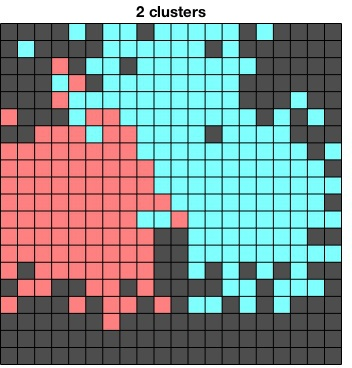The Agglomerative Information Bottleneck (AIB) algorithm greedily compresses discrete data by iteratively merging the two elements which cause the mutual information between the data and the class labels to decreases as little as possible.

Here we test AIB on the problem of finding a discriminatively optimal quantization of a mixture of Gaussians. The data in this case is 2 dimensional:Random data generated from a Gaussian mixture with three components (class labels are indicated by color).

We quantize this data on a fixed lattice (a 20x20 grid shown in the figures below), and construct histograms for each class.

f1 = quantize(X1,D,K) ;
f2 = quantize(X2,D,K) ;
f3 = quantize(X3,D,K) ;

Pcx(1,:) = vl_binsum(Pcx(1,:), ones(size(f1)), f1) ;
Pcx(2,:) = vl_binsum(Pcx(2,:), ones(size(f2)), f2) ;
Pcx(3,:) = vl_binsum(Pcx(3,:), ones(size(f3)), f3) ;


Next we apply AIB:

[parents, cost] = vl_aib(Pcx) ;


This provides us with a list of parents of each column in Pcx, forming a tree of merges. We can now "cut" this tree to obtain any number of clusters.Three "cuts" of the merge tree, showing 10, 3, and 2 clusters. The gray squares are nodes of the tree which did not have any data points which were quantized to them.

Notice that the resulting clusters do not have to be contiguous in the original space.